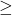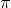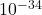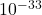## To what uncertainty (in m) can the position of a baseball traveling at 45.0 m/s be measured if the uncertainty of its speed is 0.10%? The ma

Question

To what uncertainty (in m) can the position of a baseball traveling at 45.0 m/s be measured if the uncertainty of its speed is 0.10%? The mass of a baseball is about 0.145 kg.

in progress 0
4 weeks 2021-08-18T03:48:56+00:00 2 Answers 0 views 0

Δx ≥ 8 x 10^(-29) m

Explanation:

We will solve this using Heisenberg’s Uncertainty Principle which states that one cannot simultaneously measure with great precision both the momentum, and the position of a particle.

Thus, mathematically, this is expressed as

Δp ⋅ Δx ≥ h/4π

where;

Δp is the uncertainty in momentum;

Δx is the uncertainty in position;

h is Planck’s constant which has a value of 6.626 x 10^(−34) m²kg/s

Furthermore, the uncertainty in momentum can be written as;

Δp = m ⋅ Δv

where Δv is the uncertainty in velocity while m is the mass of the particle.

In this question, the mass of the baseball is 0.145kg or 145g with an uncertainty in velocity of 0.1%

So, uncertainty in velocity = 0.1% x 45 = 0.045 m/s

Thus, the uncertainty in momentum will be;

Δp = 0.145kg x 0.045 m/s = 6.525 x 10^(-3) m.kg/s

Now, let’s plug in the relevant data into the Uncertainty Principle equation and make Δx the subject.

Thus;

Δx ≥ (h/4π) x (1/Δp)

Δx ≥ [(6.626 x 10^(−34))/(4π)] /(1/(6.525 x 10^(-3)))

= 8.08 x 10^(-29) m

If we round to one sig fig, the uncertainty in velocity, will be

Δx ≥ 8 x 10^(-29) m

Using the heisenbergs uncertainty principle equation

Δx . Δph / 4first find the speed for 0.10% : 45 m/s / 100% = x / 0.10%

∴ Δv = 0.045 m/s

Δx6.626×/ 4×× 0.145×0.045

Δx8.081×m

Explanation:

heisenbergs uncertainty principle equation allows to find the uncertainty position (in m) where one calculates the uncertainty speed of 0.10%  by simple  first identifying the uncertainty speed of 100%